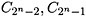Mixed Signal VLSI Wireless Design: Circuits and Systems

# 7.6: TYPES OF ANALOG-TO-DIGITAL CONVERTERS

## 7.6 TYPES OF ANALOG-TO-DIGITAL CONVERTERS

Analog-to-digital converters are classified into one-step architectures, such as: flash, folding and interpolative topologies, and multi-step architectures, such as: Successive approximation and pipeline topologies.

### 7.6.1 Flash Analog-to-Digital Converter

The flash architecture is potentially the fastest analog-to-digital converter, because of the parallelism it employs. However, this parallelism puts a practical limit on the resolution of the flash analog-to-digital converter. For an n-bit analog-to-digital converter, the flash architecture employs (2 n ?1) comparators and decoding logic. A resistive ladder consisting of 2 n equal resistors divides the reference voltage into 2 n equally spaced voltages as shown in Figure 7.15Figure 7.15: Block diagram of an n-bit flash analog-to-digital converter.

The output of the comparator constitutes a thermometer code. Assume that the analog input signal ? in is such that:

 (7.33)Therefore, the output of comparators C 1, C 2 , C i is logic 1, while the output of comparator C i +1 , C i +2 ,is logic 0. The decoding logic transfers the thermometer code which has (2 n ?1) bits into a binary signal which has n bits. Table 7.1 shows the mapping between the thermometer code and the binary coded signal when n=3.

Table 7.1: The thermometer code of a binary coded signal for n=3.

Thermometer code

Binary code

0000000

000

0000001

001

0000011

010

0000111

011

0001111

100

0011111

101

0111111

110

1111111

111